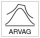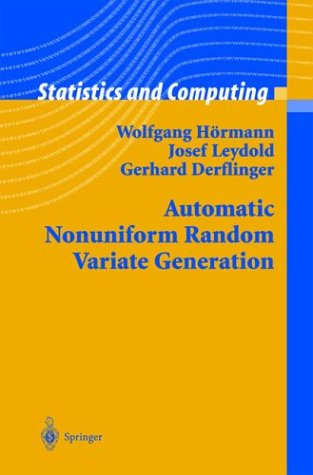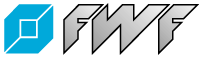ARVAG - Automatic nonuniform Random VAriate GenerationW. Hörmann, J. Leydold, and G. Derflinger Automatic Nonuniform Random Variate Generation   Series: Statistics and Computing 2004, X, 442pp, Hardcover ISBN: 3-540-40652-2 Springer, Berlin

Errata

• p.47, Line 14/15: [Thanks to Halis Sak (PhD Student, Bogazici University, Istanbul)]
... we have included two variants of Step 4.
• p.80, Sect. 4.4.4 Equiangular Points:
The parameter xi in Eq.(4.21) must be less than or equal to one. Otherwise, some of the points pi are outside the given domain (bl , br ).
Alternatively one could use thetal = arctan ((bl - m) / xi ) and analogously for thetar .
• p.94, Algorithm 4.4 (TDR 3-points): line 12 has to be completed to
12: Set Ai <-- 0,    bj <-- bj+k.

• p.122, Algorithm 5.3 (Ahrens-Equal-Area): [Thanks to Umut Sevin (PhD Student, Bogazici University, Istanbul)]
line 14: replace j by J (two times).
line 24: replace i by J (in comment).
line 27: complete to `if (X<=b_r && Y <= f(X)) then` [return X].Wolfgang Hörmann and Josef Leydold   (October 21st, 2003) Research supported by